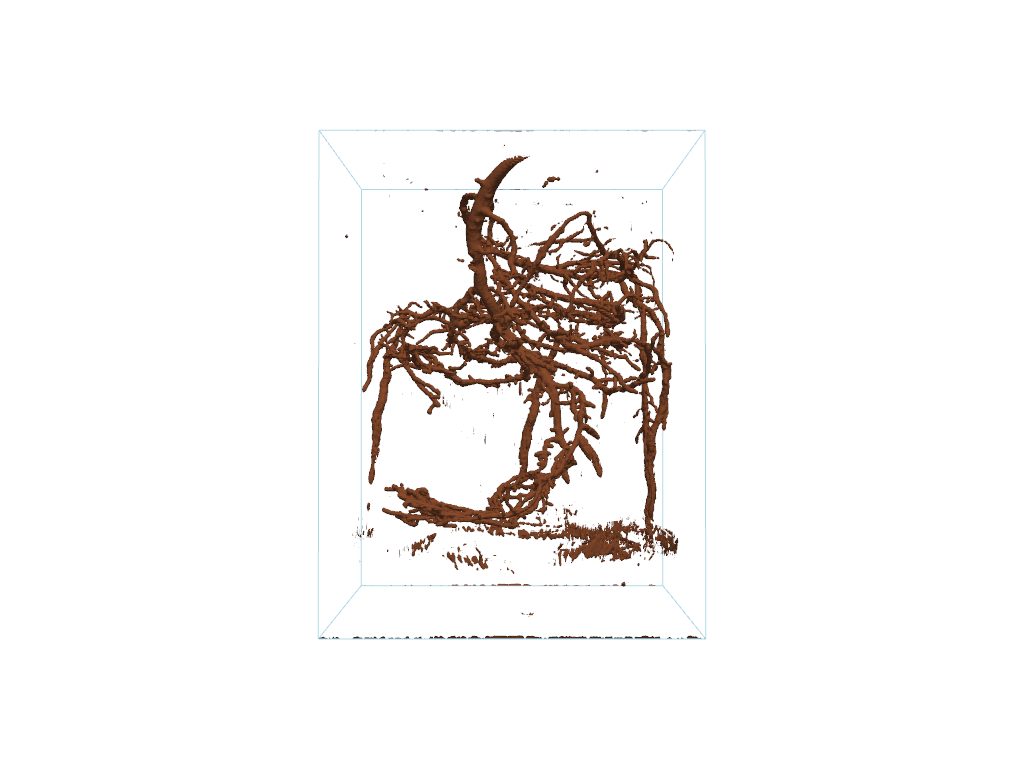# Connectivity#

Use the connectivity filter to remove noisy isosurfaces.

This example is very similar to this VTK example

```import pyvista as pv
from pyvista import examples
```

Load a dataset that has noisy isosurfaces

```mesh = examples.download_pine_roots()

cpos = [(40.6018, -280.533, 47.0172), (40.6018, 37.2813, 50.1953), (0.0, 0.0, 1.0)]

# Plot the raw data
p = pv.Plotter()
p.show(cpos=cpos)
```The mesh plotted above is very noisy. We can extract the largest connected isosurface in that mesh using the `pyvista.DataSetFilters.connectivity()` filter and passing `largest=True` to the `connectivity` filter or by using the `pyvista.DataSetFilters.extract_largest()` filter (both are equivalent).

```# Grab the largest connected volume present
largest = mesh.connectivity(largest=True)
# or: largest = mesh.extract_largest()

p = pv.Plotter()